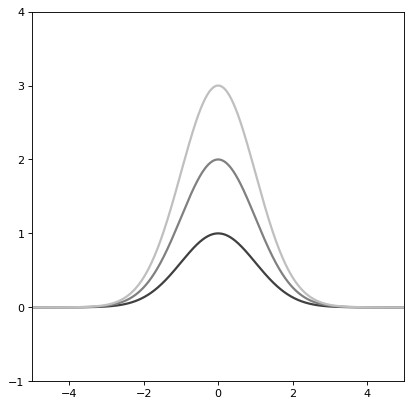# Gaussian1D¶

class astropy.modeling.functional_models.Gaussian1D(amplitude=1, mean=0, stddev=1, **kwargs)[source]

One dimensional Gaussian model.

Parameters
amplitude

Amplitude (peak value) of the Gaussian - for a normalized profile (integrating to 1), set amplitude = 1 / (stddev * np.sqrt(2 * np.pi))

mean

Mean of the Gaussian.

stddev

Standard deviation of the Gaussian with FWHM = 2 * stddev * np.sqrt(2 * np.log(2)).

Other Parameters
fixeda dict, optional

A dictionary {parameter_name: boolean} of parameters to not be varied during fitting. True means the parameter is held fixed. Alternatively the fixed property of a parameter may be used.

tieddict, optional

A dictionary {parameter_name: callable} of parameters which are linked to some other parameter. The dictionary values are callables providing the linking relationship. Alternatively the tied property of a parameter may be used.

boundsdict, optional

A dictionary {parameter_name: value} of lower and upper bounds of parameters. Keys are parameter names. Values are a list or a tuple of length 2 giving the desired range for the parameter. Alternatively, the min and max properties of a parameter may be used.

eqconslist, optional

A list of functions of length n such that eqcons[j](x0,*args) == 0.0 in a successfully optimized problem.

ineqconslist, optional

A list of functions of length n such that ieqcons[j](x0,*args) >= 0.0 is a successfully optimized problem.

Notes

Either all or none of input x, mean and stddev must be provided consistently with compatible units or as unitless numbers.

Model formula:

$f(x) = A e^{- \frac{\left(x - x_{0}\right)^{2}}{2 \sigma^{2}}}$

Examples

>>> from astropy.modeling import models
>>> def tie_center(model):
...         mean = 50 * model.stddev
...         return mean
>>> tied_parameters = {'mean': tie_center}


Specify that ‘mean’ is a tied parameter in one of two ways:

>>> g1 = models.Gaussian1D(amplitude=10, mean=5, stddev=.3,
...                             tied=tied_parameters)


or

>>> g1 = models.Gaussian1D(amplitude=10, mean=5, stddev=.3)
>>> g1.mean.tied
False
>>> g1.mean.tied = tie_center
>>> g1.mean.tied
<function tie_center at 0x...>


Fixed parameters:

>>> g1 = models.Gaussian1D(amplitude=10, mean=5, stddev=.3,
...                        fixed={'stddev': True})
>>> g1.stddev.fixed
True


or

>>> g1 = models.Gaussian1D(amplitude=10, mean=5, stddev=.3)
>>> g1.stddev.fixed
False
>>> g1.stddev.fixed = True
>>> g1.stddev.fixed
True

import numpy as np
import matplotlib.pyplot as plt

from astropy.modeling.models import Gaussian1D

plt.figure()
s1 = Gaussian1D()
r = np.arange(-5, 5, .01)

for factor in range(1, 4):
s1.amplitude = factor
plt.plot(r, s1(r), color=str(0.25 * factor), lw=2)

plt.axis([-5, 5, -1, 4])
plt.show()


(png, svg, pdf)Attributes Summary

 amplitude fwhm Gaussian full width at half maximum. input_units This property is used to indicate what units or sets of units the evaluate method expects, and returns a dictionary mapping inputs to units (or None if any units are accepted). mean param_names Names of the parameters that describe models of this type. stddev

Methods Summary

 evaluate(x, amplitude, mean, stddev) Gaussian1D model function. fit_deriv(x, amplitude, mean, stddev) Gaussian1D model function derivatives.

Attributes Documentation

amplitude = Parameter('amplitude', value=1.0)
fwhm

Gaussian full width at half maximum.

input_units
mean = Parameter('mean', value=0.0)
param_names = ('amplitude', 'mean', 'stddev')

Names of the parameters that describe models of this type.

The parameters in this tuple are in the same order they should be passed in when initializing a model of a specific type. Some types of models, such as polynomial models, have a different number of parameters depending on some other property of the model, such as the degree.

When defining a custom model class the value of this attribute is automatically set by the Parameter attributes defined in the class body.

stddev = Parameter('stddev', value=1.0, bounds=(1.1754943508222875e-38, None))

Methods Documentation

static evaluate(x, amplitude, mean, stddev)[source]

Gaussian1D model function.

static fit_deriv(x, amplitude, mean, stddev)[source]

Gaussian1D model function derivatives.